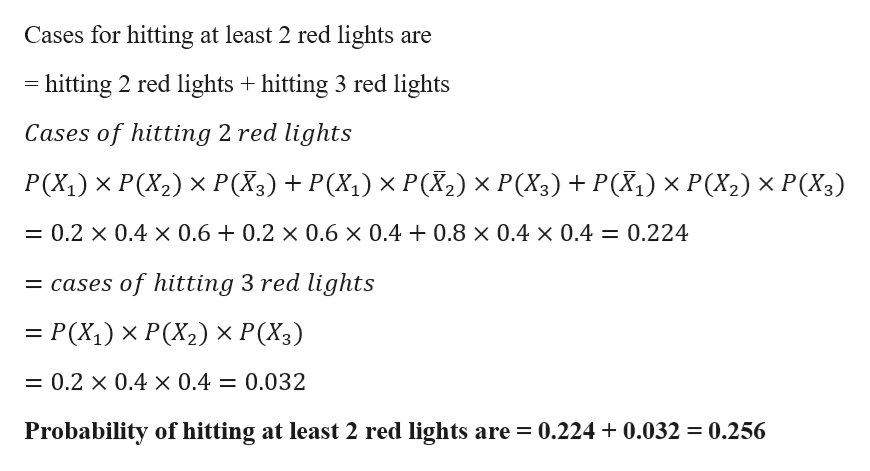# A Driver keeps goes through 3 intersections. The probabibilty of hitting a red light at each are 0.2, 0.4, and 0.4 respectively. What is the probabibility of hitting at least two red lights?

Question
24 views

A Driver keeps goes through 3 intersections. The probabibilty of hitting a red light at each are 0.2, 0.4, and 0.4 respectively. What is the probabibility of hitting at least two red lights?

check_circle

Step 1

Let xi denotes the probability of hitting red light in ith intersections
G...help_outlineImage TranscriptioncloseCases for hitting at least 2 red lights are hitting 2 red lights hitting 3 red lights Cases of hitting 2 red lights Р(X) x Р(Х2) х Р(Х3) + P(X,) x Р(X) x Р(Х3) + P(X,) Х Р(X2) х Р(Х3) = 0.2 x 0.4 x 0.6 + 0.2 x 0.6 x 0.4 + 0.8 x 0.4 x 0.4 = 0.224 of hitting 3 red lights = cases — Р(X) х Р(Х2) Х P(X3) = 0.2 x 0.4 x 0.4 = 0.032 Probability of hitting at least 2 red lights are = 0.224 0.032 =0.256 fullscreen

### Want to see the full answer?

See Solution

#### Want to see this answer and more?

Solutions are written by subject experts who are available 24/7. Questions are typically answered within 1 hour.*

See Solution
*Response times may vary by subject and question.
Tagged in

### Basic Probability